Select Page

# MCQ Determinant CBSE Maths 12 Science Solutions in English

MCQ Determinant CBSE Maths 12 Science Solutions in English to enable students to get Solutions in a narrative video format for the specific question.

Expert Teacher provides MCQ Determinant CBSE Maths 12 Science Solutions through Video Solutions in English language. This video solution will be useful for students to understand how to write an answer in exam in order to score more marks. This teacher uses a narrative style for a question from Determinant not only to explain the proper method of answering question, but deriving right answer too.

Please find the question below and view the Solution in a narrative video format.

Question:

Solution Video in English:

You can select video Solutions from other languages also. Please check Solutions in ( Hindi )

## Similar Questions from CBSE, 12th Science, Maths, Determinant

Question 1 :  Find the area of the triangle with vertices at the points (2, 7), (1, 1), (10, 8). (View Answer Video)

Question 2 : Evaluate the determinant: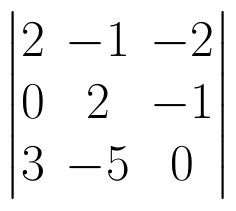. (View Answer Video)

Question 3 :  If A is a square matrix of order 3 and |A| = 5 then |3A| = ? (View Answer Video)

Question 4 : If |A| = 0, then A is, (View Answer Video)

Question 5 : Find the adjoint  of the matrix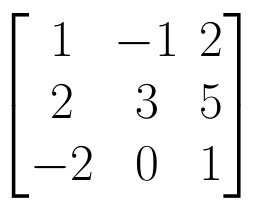. (View Answer Video)

### Application of Integrals

Question 1 : Using integration find the area of the triangular region whose sides have the equations y = 2x + 1, y = 3x + 1 and x = 4. (View Answer Video)

Question 2 : Using the method of integration, find the area of the region bounded by the lines 3x - y - 3 = 0, 2x + y - 12 = 0 and x -2y - 1 = 0. (View Answer Video)

Question 3 : Find the area of the circle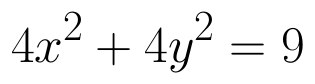which is interior to the parabola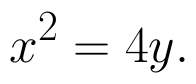(View Answer Video)

Question 4 : Find the area of the given curves and given lines: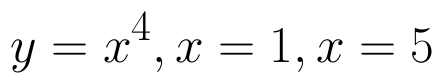and x-axis (View Answer Video)

Question 5 : Find the area enclosed by the parabola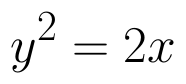and the line x - y = 4. (View Answer Video)

### Inverse Trigonometric Functions

Question 1 : Write the principal value of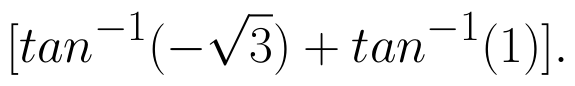(View Answer Video)

Question 2 : Using principal values, write the value of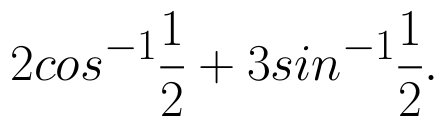(View Answer Video)

Question 3 : Write the value of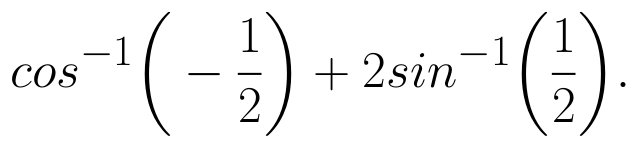(View Answer Video)

Question 4 : Write the principal value of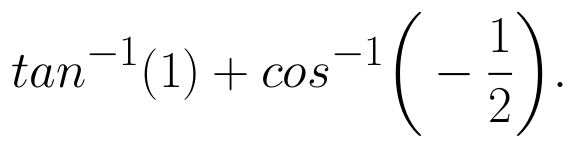(View Answer Video)

Question 5 : Solve for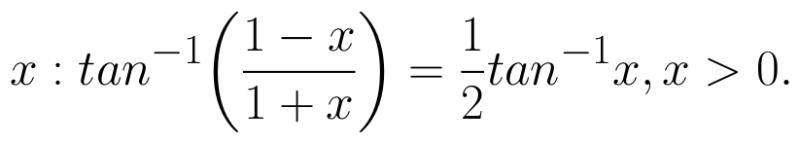(View Answer Video)

### Application of Derivatives

Question 1 : The slope of the normal to the curve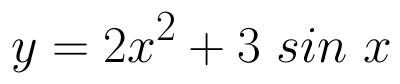at x = 0 is: (View Answer Video)

Question 2 : Find the approximate change in the surface area of a cube of side x meters caused by decreasing the side by 1%. (View Answer Video)

Question 3 : Equation of normal to the curve x+y=x^y where it cuts x-axis; is (View Answer Video)

Question 4 : The slope of the normal to the curve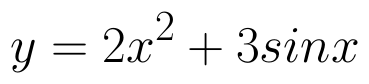at x = 0 is :
Question 5 : Find two positive numbers x  and y such that x+y=60 and is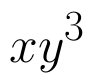maximum. (View Answer Video)